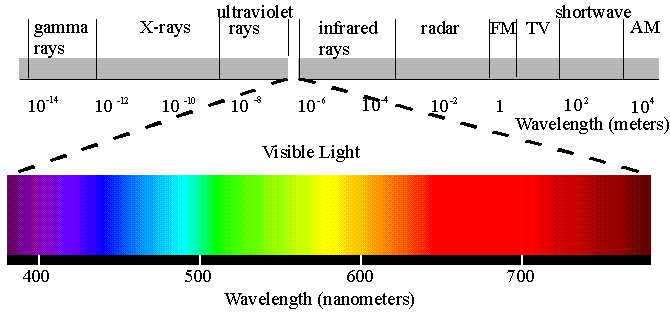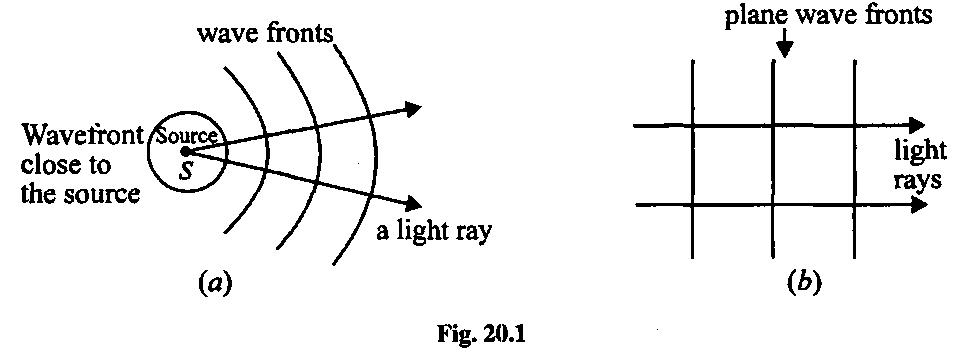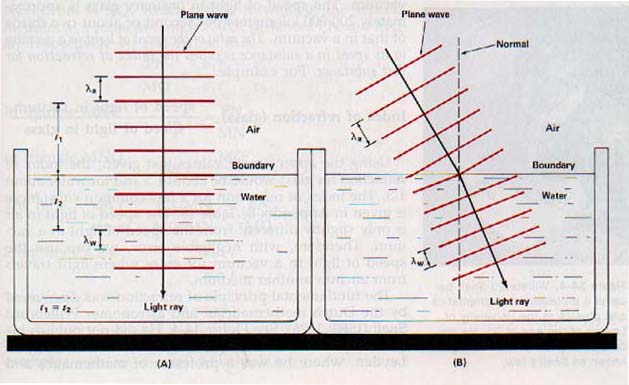# Lecture 23: Light: wavefronts, rays, reflection and refraction

## Properties of light

• Light is electromagnetic (EM) phenomena, electromagnetic radiation

## Light as electromagnetic wave

• In classical (i.e not quantum) picture the light is a wave of oscillating interconnected electric and magnetic fields
• EM wave is not a mechanical wave.
• EM phenomena are governed by Maxwell equation , which, in particular, show that at every instance in electromagnetic wave in vacuum electric field E and magnetic field H are perpendicular to each other.

• EM wave in vacuum has a speed c = 2.99792458 × 108 m/s .
Speed of light in vacuum is a fundamental physical constant
• EM waves can have different frequencies and wavelength. Distribution of light in frequency (wavelength) is EM spectrum . People identify several region in EM spectrum. although basic nature of radiation is the sameAlthough there is no limit to a wavelength, useful wavelengths range from kilometers in for radio waves to 10-14 m for X-rays. Visible light lies in the narrow range of wavelengths around 400-600 nanometers = 4 - 6 × 10-7 meters (fraction of a micron)

## Light as a particile

• In 19th and early 20th century discovery of unusual properties of light lead to revolution in physics and development of Special Relativity and Quantum Mechanics
• Speed of light in vacuum was found to be constant independently on the reference frame. Quite contrary to Newtonian mechanics this lead to Special Relativity (by Albert Einstein, Hendrik Lorentz, Henri Poincare ) which incorporates constancy of the speed of light as a fundamental principle.
• Speed of light in vacuum is the highest velocity a material body can attain, so it is the highes velocity information can be transmitted.
• Then it was discovered that light is emitted and absorbed not as a continous wave, but in packets, "quanta". This explained (by Max Planck) the spectrum of radiation by 'black bodies' (perfect thermal emitters/absorbers) and led to Quantum Mechanics
• When quantum phenomena manifests itself (as in absorption and emission), light presents itself a sequence of particle (photons) which have energy proprtional to the frequency E = ℏ f . is called the Planck's constant and is another fundamental constant of nature.

## Wavefronts and rays

• Ray is a line everywhere perpendicular (normal) to the surfaces of the constant phase of the wave - wavefronts. Let us draw some on the board
• Rays point in a local direction of the propagation of the wave.
• To draw a family of rays is another way to represent the wave propagation
• In a plane wave all rays are parallel to each other, and one can track just a single ray.
• In spherical wave rays are along the radial directions and are divergent.• In more complex waves rays can intersect
• In optics it is sufficient to track just the rays, if one is inetrested in phenomena over distances larger than a wavelength of light. This is approximation of geometrical optics
• Rays should not be confused with beams of light. Ray is an imaginary line that points to the direction of wave propagation, beam is a physical light that has intensity over a finite cross-section. The wave in a beam can be nearly plane-parallel (good laser) in which case the beam will have almost finite cross-section over large distances, or not, in which case beam will diverge in a conical fashion. Plane-parallel light in the beam is well described by a single ray direction, while divergent beam is not (one uses pencil of rays to describe such beams)

## index of refraction

• In the material the light always moves with speed v that is slower than in vacuum.
• The ration n = c/v is called index of refraction and is one of the most important optical properties of a material. Typical values for glasses are n ~ 1.5-2 while for the air it is 1.0003, which is practically unity
• Index of refraction is always larger than unity n > 1 , equal to unity only in vacuum.

## Refraction and reflection

Two fundamental optical phenomena - reflection and refraction - happen at the interface between two materials with different index of refraction n
• Part of the light is reflected. Ultimate example is the mirror, where practically all the light is reflected  The law of reflection: angle between the incident ray and the normal to the surface is the same as the angle between reflected ray and the normal θr = θa
• Part of the light is transmitted into another material, exhibiting refraction - change of direction of propagation  The law of refaction: angle between the incident ray and the normal to the surface and the angle between transmitted ray and the normal are in relation na sin θa = nb sin θb The angle is smaller in the material with higher refractive index ( nb at the bottom )

## Brief explanation of refraction effect